﻿ Using Gamma-Ray to Determine the Homogeneity of Some Building Materials

### Using Gamma-Ray to Determine the Homogeneity of Some Building Materials

Laith A. Najam, Ahmed K. Mheemeed, Isra.a M. HassanOPEN ACCESSPEER-REVIEWED

## Using Gamma-Ray to Determine the Homogeneity of Some Building Materials

Laith A. Najam1,, Ahmed K. Mheemeed2, Isra.a M. Hassan1

1Department of Physics, College of Science, University of Mosul, Iraq

2Department of Physics, College of Education, University of Mosul, Iraq

### Abstract

In this paper, we use a narrow collimated beam of mono- energetic gamma rays emitted from the radioactive source 137Cs (662 keV) and the system of measuring gamma rays UCS-20 associated with the sodium iodide detector NaI (Tl) to determine the homogeneity of the building materials, Granite, Marble, Cement, Ceramic and Bricks. The homogeneity test was determined from the percentage difference between the transmitted intensity of gamma-rays and the linear attenuation coefficient at different positions for each sample of the material then the percentage of standard deviation was checked.

### At a glance: Figures

12
Prev Next

• Najam, Laith A., Ahmed K. Mheemeed, and Isra.a M. Hassan. "Using Gamma-Ray to Determine the Homogeneity of Some Building Materials." International Journal of Physics 2.1 (2014): 23-29.
• Najam, L. A. , Mheemeed, A. K. , & Hassan, I. M. (2014). Using Gamma-Ray to Determine the Homogeneity of Some Building Materials. International Journal of Physics, 2(1), 23-29.
• Najam, Laith A., Ahmed K. Mheemeed, and Isra.a M. Hassan. "Using Gamma-Ray to Determine the Homogeneity of Some Building Materials." International Journal of Physics 2, no. 1 (2014): 23-29.

 Import into BibTeX Import into EndNote Import into RefMan Import into RefWorks

### 1. Introduction

Nuclear radiation is used in many fields including nuclear physics, medical physics, physics, health, agriculture, industry and other. These rays are used in industry for measuring the rate of flow of gases and liquids in pipelines and to study the friction between the surfaces, determine the amount of corrosion in the spare machines and, motors, control of the thickness of the plates made of metal and plastic, measure the density of liquids and pastes, and checking the homogeneity of materials and others. The homogeneity of thin films, plastics and rubbers can be studied through the variation in the energy, intensity and attenuation coefficient of nuclear radiation by using definite charge particles such as electrons, protons and alpha particles . Beta particles emitted from the radioactive source 90Sr [2, 3] were used for measuring the homogeneity of mix rubber type MCG used in masks prevention. Electromagnetic radiations, X-ray and γ-ray can be used in determining the degree of homogeneity of aluminum, iron, lead, alloys and other substances with high density. Technology use transmission gamma rays (GRT) and X-ray micro tomography (μ-CT)  to detect the presence of pores inside the material and determine the degree of homogeneity of the material. Gamma-rays emitted from radioactive sources 137Cs, 152Eu and 241Am are used for measuring the homogeneity of set of samples produced by mixing lead with a rubber type NBR with different weight ratios. The homogeneity of these samples was studied through the variation in the transmitted intensity of gamma-rays and their linear attenuation coefficient.

As the usage of γ-ray increased in various fields from medical applications to food industry, it became important to get protection from their detrimental effects. There are three general rules of protections: time, distance and shielding. In most cases, shielding is the main rule to be performed . The attenuation coefficient is important parameter for studying interaction of γ-ray with matter that gives us the fraction of energy scattered or absorbed. The attenuation coefficient can be extensively found in the literature. Many authors have studied γ-ray attenuation coefficient in shielding materials [5, 7, 8, 9, 10].

The building materials used in our markets are different in their homogeneity, so we used gamma rays emitted from the radioactive source 137Cs and the system of measuring gamma rays UCS-20 associated with the sodium iodide detector NaI (Tl) in the examination of the homogeneity of building materials used in Nineveh governorate (Iraq). The linear attenuation coefficients for the building materials were determined

### 2. Experimental Method

In the present study, 16 samples of building materials were collected from the local markets at Nineveh governorate, some are produced inside the country and others are imported. These materials are Granite, Marble, Cement, Ceramic and Bricks. The homogeneity test of these materials were determined by studying the variations in the transmitted intensity of gamma-rays at four positions for each sample of the studied materials, linear attenuation coefficient and the percentage of standard deviation. The homogeneity test was performed by using the gamma ray system UCS-20 associated with the sodium iodide detector NaI (Tl) with dimensions 2.5cm × 3.8 cm shown in Figure 1. This system consists of preamplifier, amplifier, power supply, multi channel analyzer and a personal computer for the purpose of operating, reading measurements and analysis of the results. A narrow collimated beam of mono- energetic gamma rays emitted from the radioactive source 137Cs (662 keV) was obtained using a lead Cylinder with height (70 mm), diameter (60 mm) and an internal hole with diameter (4mm). The sample was placed inside a slot with width equal to the sample thickness (10mm, 20mm) as shown in Figure 1.

### 3. Calculations

3.1. Variation of Intensity

When the narrow beam of mono- energetic gamma rays is incident with intensity Iο at a material medium, the intensity of transmitted rays I is  :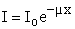(1)

Where: μ is the linear attenuation coefficient which depends on the energy of gamma rays and the components of the material medium.

The homogeneity of the material can be known by comparing the intensity of transmitted gamma radiation I through identified sites in the material with average values of I' for all positions. Besides we can determine the percentage of the homogeneity of material ΔI (%) by using the following relationship: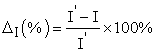(2)
3.2. Variation of Linear Attenuation Coefficient

The linear attenuation Coefficient of gamma rays μ has been calculated by using equation (1). The homogeneity of the material can be worked out by comparing the values of μ in the specific locations in the material with the average values of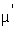. The percentage of the homogeneity of material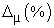is given by: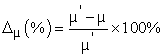(3)
3.3. Percentage of Standard Deviation

The values of the standard deviations were calculated using the following formulae.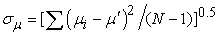(4)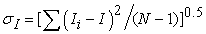(5)

Where:

σ is the standard deviation

N=4 is the number of measured positions of each sample.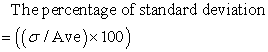(6)

Whenever the values of ΔI (%),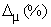and ((σ / Ave) × 100) do increase, it means that the homogeneity of materials does increase .

### 4. Results and Discussion

Table 1 to Table 5 contain the values of IO, ρ, x, p, I, μ, ΔI(%) and Δµ(%) at four positions for each sample of the studied materials, Granite, Marble, Cement, Ceramic and Bricks. The values of I',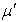,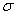and the percentage values of standard deviation ((σ / Ave) × 100) are also illustrated in these tables. The relationship between each of ΔI(%) and Δµ(%) at four positions of the studied materials are shown in Figure 2 to Figure 6. We note from these tables and figures that the positive values of ΔI(%) corresponde to negative values of Δµ(%), which means that any decrease in μ offset is caused by an increase in I at any position and vice versa. It is clear from Table 1 and Figure 2 that the minimum and maximum values of ΔI(%) and Δµ(%) have been obtained in Turkish and Chinese Granite, respectively. The lower and higher percentage values of standard deviation ((σI / I') × 100) and ((σμ /) × 100) were 1.61%, 4.01% and 4.04%, 9.28% and found in Turkish and Chinese Granite, respectively. The Turkish Granite is more homogeneous than the Chinese Granite. Figure 3 and Table 2 show that the lowest and highest values of ΔI(%) and Δµ(%) were detected in Turkish and Spanish Marble, respectively. The lower and higher percentage values of standard deviation ((σI / I') × 100) and ((σμ /) × 100) were 1.283%, 3.55% and 1.61%, 4.84% and found in Turkish and Spanish Marbles. The Turkish Marble is more homogeneous than the Spanish Marble. The highest and lowest values of ΔI(%) and Δµ(%) were found in Iraqi and Turkish Cement, respectively and shown in Table 3 and Figure 4. The higher and lower percentage values of standard deviation ((σI / I') × 100) and ((σμ /) × 100) were 1.73%, 5.0% and 1.57%, 4.35% and found in Iraqi and Turkish Cement, respectively. The Turkish Cement is more homogeneous than the Iraqi Cement. It is clear from Table 4 and Figure 5 that the minimum and maximum values of ΔI(%) and Δµ(%) have been obtained in Spanish and Italian Ceramic, respectively. The lower and higher percentage values of standard deviation ((σI / I') × 100) and ((σμ /) × 100) were 0.539%, 3.47% and 0.556%, 3.8% and found in Spanish and Italian Ceramic, respectively. The Spanish Ceramic is more homogeneous than the Italian Ceramic. We found from Figure 6 and Table 5 that the lowest and highest values of ΔI(%) and Δµ(%) were detected in Iranian and Iraqi Bricks, respectively. The lower and higher percentage values of standard deviation ((σI / I') × 100) and ((σμ /) × 100) were 0.733%, 2.06% and 0.938%, 3.12% and found in Iranian and Iraqi Bricks. The Iranian Brick is more homogeneous than the Iraqi Brick. Current results show that the Spanish Ceramic sample is more homogeneous while the Chinese Granite sample is less homogeneous as compared with the other building materials

#### Table 5. The results of homogeneity tests of Brick material

Figure 6. The relationship between each ΔI(%) and Δµ(%) with the position for Brick material

The average values of linear attenuation Coefficient of gamma rays varies from o.218 cm-1 (Spanish Granite) to o.159 cm-1 (Iraqi Brick). The average values of linear attenuation Coefficient were 0.206 cm-1, 0.194 cm-1, 0.192 cm-1, 0.171 cm-1 and 0.16 cm-1 for building materials, Granite, Marble, Cement, Ceramic and Bricks, respectively. Current results have shown that Granite is one of the best building materials to be used in shielding against gamma rays, whereas Bricks showed less ability to attenuate gamma rays, because Bricks density is low as compared with the Granite density. The current values of μ for Granite material ranged from 0.198 cm-1 to 0.218 cm-1 are comparable with those reported by Mavi  and higher than the value of μ of known shielding materials, for example Concrete (white sand) , Diorite rock  and compressed soil .

### 5. Conclusions

The building materials used in our markets are different in their homogeneity, so we used a narrow collimated beam of mono- energetic gamma rays to test the homogeneity of materials. The transmitted intensity of gamma-rays at different positions for each sample ΔI (%), linear attenuation coefficient Δµ(%) and the percentage of standard deviation ((σ / Ave) × 100) were used in the examination of the homogeneity of materials. It was observed from the values of ΔI (%), Δµ(%) and ((σ / Ave) × 100) shown in Table 1 to Table 5 and Figure 2 to Figure 6 that the Spanish Ceramic sample is more homogeneous while the Chinese Granite sample is less homogeneous as compared with the other building materials. Current results have shown that Granite is one of the best building materials to be used in shielding against gamma rays.

### References

  Lilley J (2001) Nuclear physics principles and application. Wiley, University of Manchester, New York.In article  Suleiman WA, Ali S A (2005) Using beta-particle for measuring the homogeneity of rubber compound and studying the effect of compression, temperature and pressure on its homogeneity and mechanical properties. Raf J Sci 16(1):115-121.In article  Ali S A, Suleiman W A (2006) The effect of mixing time on the homogeneity and mechanical specifications for rubber mixtures. Raf J Sci 17(1): 103-110.In article  Moreir A C, Appoloni C R, Oliveira M V, Fernandes C P (2007) Structural Characterization of Titanium Porous Foams by Gamma Ray Transmission and X-Rays Microtomography, International Nuclear Atlantic Conference, INAC Sontos, SP, Brazel September 30 to October 5.In article  Mheemeed A K, Hasan I H, Al-Jomaily F M(2012) Gamma-ray absorption using rubber-lead mixtures as radiation protection shields. J Radioanal Nucl Chem 291:653-659.In article CrossRef  Eaves G (1964) Principles of radiation protection, Iliffe Books Ltd, London.In article  Mavi B (2012) Experimental investigation of γ-ray attenuation coefficients for granites. Annals of Nuclear Energy 44:22-25.In article CrossRef  Jameel H, Al-dayel O, Al-horayess O, Al-ajyan T (2010) Gamma ray shielding from white sand. Energy Power Eng 2(1): 6-9.In article CrossRef  El-Taher A (2007) Comparative study of attenuation and scattering of gamma-rays through two intermediate rocks. Indian J Pure Appl Phys 45:198-203.In article  Murbut A, Namah A, Taki A (2000) Measurement of gamma-ray attenuation in compressed soil sample. Sci J Iraqi At Energy Comm 2(2):46-52.In article